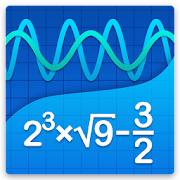# Graphing Calculator + Math, Algebra & CalculusEveryone
83,579
Graphing Calculator by Mathlab is a scientific graphing calculator integrated with algebra and is an indispensable mathematical tool for students from high school to those in college or graduate school, or just anyone who needs more than what a basic calculator offers. It is designed to replace bulky and costly handheld graphing calculators and works on virtually any Android phone or tablet.

Video: https://youtu.be/6BR8Lv1U9kA
Help site with instructions and examples: http://help.mathlab.us
If you have a question, send email to calc@mathlab.us

SCIENTIFIC CALCULATOR
• Square root, cube and higher roots (hold √ key)
• Exponent or power, use x^ key, (x^2)
• Logarithms ln(), log(), log[base]()
• Trigonometric functions sin π/2, cos 30°, ...
• Hyperbolic functions sinh, cosh, tanh, ... (hold "e" key to switch)
• Inverse functions (hold direct function key)
• Complex numbers, all functions support complex arguments
• Derivatives sin x' = cos x, ... (hold x^ key)
• Scientific and engineering notation (enable in menu)
• Percent mode
• Binary, octal and hexadecimal numbers, 0b1010, 0o123, 0xABC

GRAPHING CALCULATOR
• Multiple functions graphing
• Implicit functions up to 2nd degree (ellipse 2x^2+3y^2=1, etc.)
• Polar graphs (r=cos2θ)
• Parametric functions, enter each on new line (x=cos t, y=sin t)
• Function roots and critical points.
• Graph intersections
• Tracing function values and slopes
• Slide to scroll
• Pinch to zoom
• Fullscreen graphs (PRO)
• Function tables
• Save graphs as images
• Save tables as csv
• 3D graphs (PRO)

FRACTION CALCULATOR
• Simple and complex fractions 1/2 + 1/3 = 5/6
• Mixed numbers, use space to enter values 3 1/2
• Use parentheses (1+2)/(3+4)=3/7

ALGEBRA CALCULATOR
• Linear equations x+1=2 -> x=1
• Quadratic equations x^2-1=0 -> x=-1,1
• Approximate roots of higher polynomials
• Systems of linear equations, write one equation per line, x1+x2=1, x1-x2=2
• Polynomial long division
• Polynomial expansion, factoring

PROBABILITY AND STATISTICS
• Hold n! key to enter functions, double tap dot to enter comma
• Combinations nCr(5,2)=10
• Permutations nPr(5,2)=20
• Sample A=[1 2 3 4 5]
• Sample B=[2 3 4 5 6]
• Sum sum(A) = 15
• Average avg(A) = 3
• Median median(A) = 3
• Sample variance var(A) = 2.5
• Population variance varp(A) = 2
• Standard deviation stdev(A) = 1.58..., stdevp(A) = 1.41...
• Covariance cov(A,B) = 2.5
• Correlation corr(A,B) = 1

MATRIX CALCULATOR
• Matrix and vector arithmetic operations
• Vector cross product, dot product (hold *) and norm
• Matrix determinant, inverse, transpose and trace functions

LIBRARY
• Physical constants (PRO)
• User defined constants and functions (PRO)
• Save expressions for future reference
Collapse

Review Policy
4.6
83,579 total
5
4
3
2
1

## What's New

Chromebooks support (new Chromebooks with Google Play)
Android 8.1 support
Output format for complex numbers, Settings > Calculator

Exact values for trigonometric functions sin(60°) = 1/2*sqrt(3)
Polar form for complex numbers 1∠30°, hold i to enter ∠
Visual editing (beta), enable in Settings > Calculator

New permissions are for optional video ads.
You can choose to watch video ads to earn PRO time.
Collapse

Updated
March 28, 2018
Size
6.7M
Installs
5,000,000+
Current Version
4.14.159
Requires Android
4.1 and up
Content Rating
Everyone
Interactive Elements
Digital Purchases
Permissions
Offered By
Mathlab Apps, LLC
Developer
PO Box 701004 San Jose, CA 95170 United States